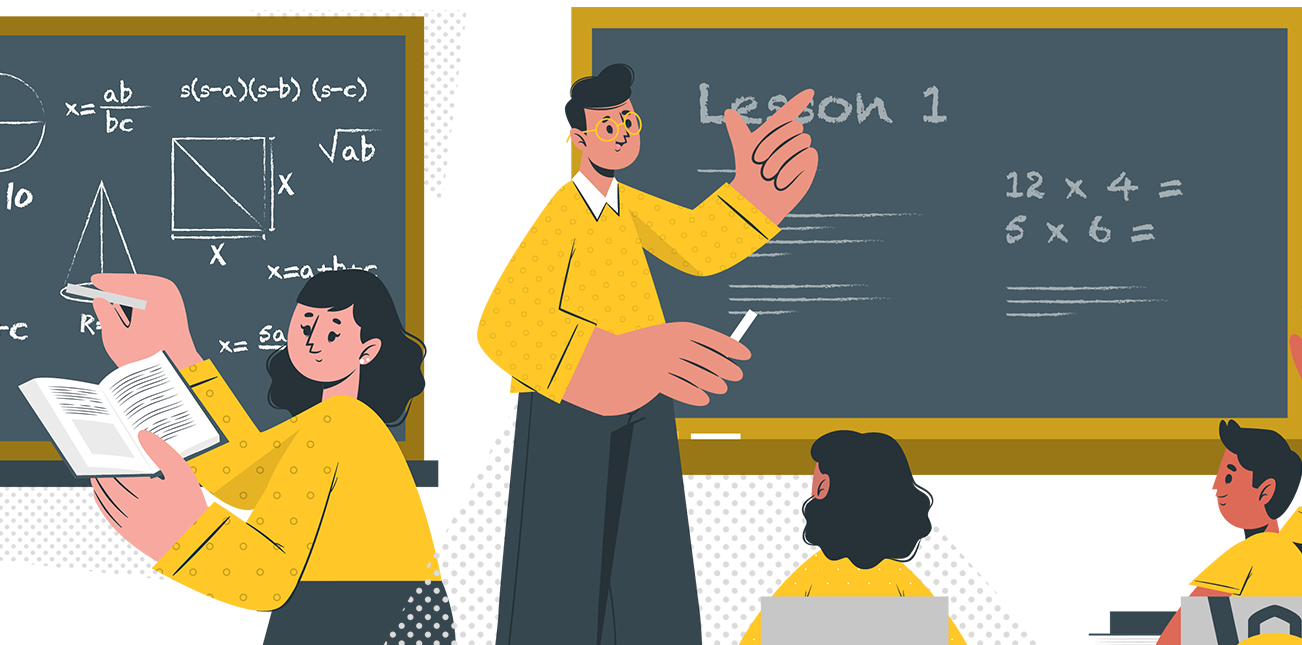# COURSE OVERVIEW

Boost your math scores! From algebra and geometry to calculus and statistics, we've got you covered.
Get tutors for homework help, study and test preparation in any math subject.

## COURSE OVERVIEW

From solving Algebra 1 and 2 problems to interpreting algebraic expressions to understanding the basics of geometric
progression, our math tutors are here to help. Get help with specific math problems from school.## MATH HOMEWORK UNTANGLED

Getting help with math homework is easy with us at Talking Chalks. With one on one interactive sessions with our tutors, solve all your math problems step by step in a real time classroom, until your homework is finished.

## WHAT TO EXPECT ?

Get a math tutor in any math subject from highschool. Whether you're still learning the basics or mastering more
difficult concepts, we're ready to help. Here are just a few of the things you can work on with a math tutor: